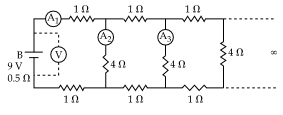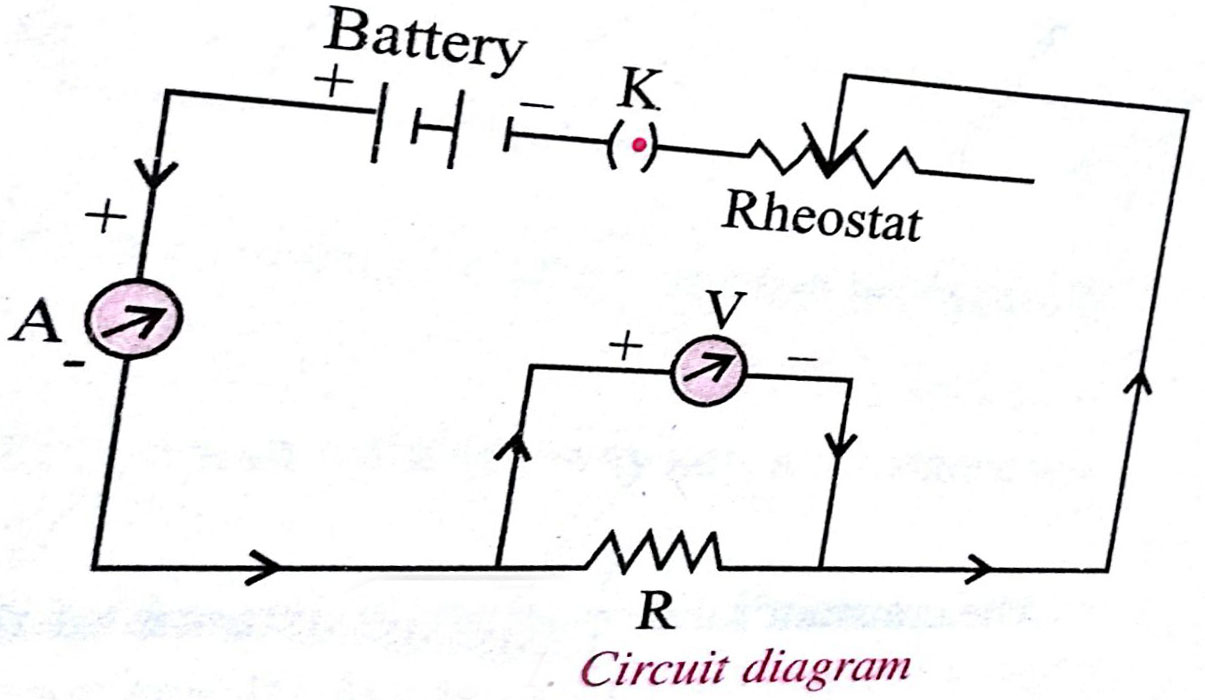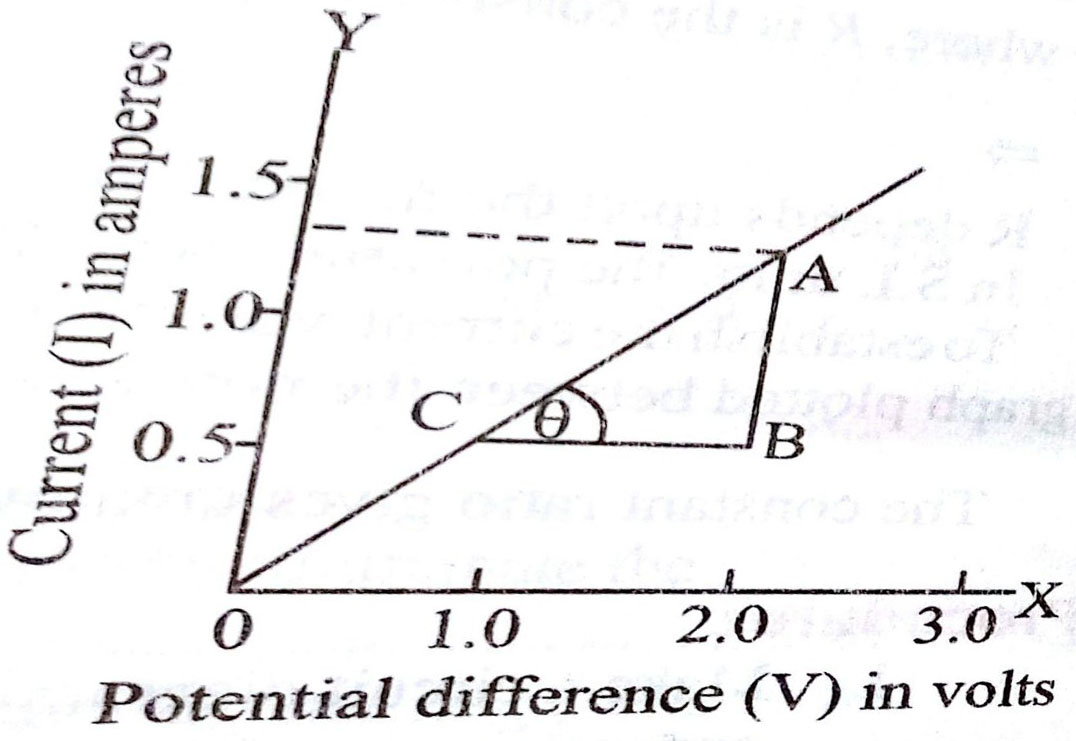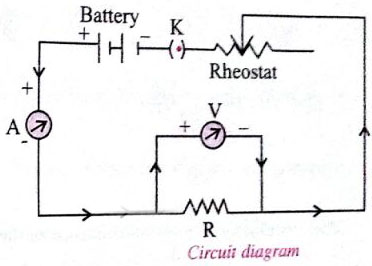#A 9 V battery with internal resistance of 0.5 is connected across an infinite network as shown in the figure.  All ammeters A1, A2, A3 and voltmeter V are ideal.Choose correct statement. Option 1)  Reading of A1 is 2 A Option 2)  Reading of A1 is 18 A Option 3)  Reading of V is 9 V   Option 4)  Reading of V is 7 V

As we learnt in

To determine resistance of given wire by plotting graph between V versus I -V= IR

R= Resistance

I = Current

v= Voltage

- whereinProcedure:

1.    Make circuit diagram as shown in the figure and arrange all the apparatus according to it.

2.    Clean the ends of the connecting wires with the help of sand paper.

3.    Find out the least counts of voltmeter and ammeter and determine the zero error.

4.    Plug the key K and slide the rhestat contact and see that ammeter and voltmeter are working properly.

5.    Adjust thesliding contact of the rheostat such that a small current passes through the resistance wire.

6.    Note down the value of potential difference V from voltmeter and current I from ammeter.

7.    Remove the key K and allow it to cool, if heated.

8.    Shift the rhestat contact slightly so that both ammeter and voltmeter show full divisions reading and not in fraction.

9.    Record the  readings of the voltmeter and ammeter.

10.    Repeat step 8 for four different settings of the rhestat. Record your observations in a tabular from and find the ratio of V and I for each set of observations.

11.    Plot a graph between potential difference V and current I taking V along X-axis and I along Y-axis. The graph comes to be a straight line as shown in figure.

12.    Cut the resistance wire at the points where it leaves the terminals, stretch it and find its length by the meter scale.

Option 1)

Reading of A1 is 2 A

This option is correct

Option 2)

Reading of A1 is 18 A

This option is incorrect

Option 3)

Reading of V is 9 V

This option is incorrect

Option 4)

Reading of V is 7 V

This option is incorrect

### Preparation Products

##### JEE Main Rank Booster 2021

This course will help student to be better prepared and study in the right direction for JEE Main..

₹ 13999/- ₹ 9999/-
##### Knockout JEE Main April 2021 (Easy Installments)

An exhaustive E-learning program for the complete preparation of JEE Main..

₹ 4999/-
##### Knockout JEE Main April 2021

An exhaustive E-learning program for the complete preparation of JEE Main..

₹ 22999/- ₹ 14999/-
##### Knockout JEE Main April 2022

An exhaustive E-learning program for the complete preparation of JEE Main..

₹ 34999/- ₹ 24999/-